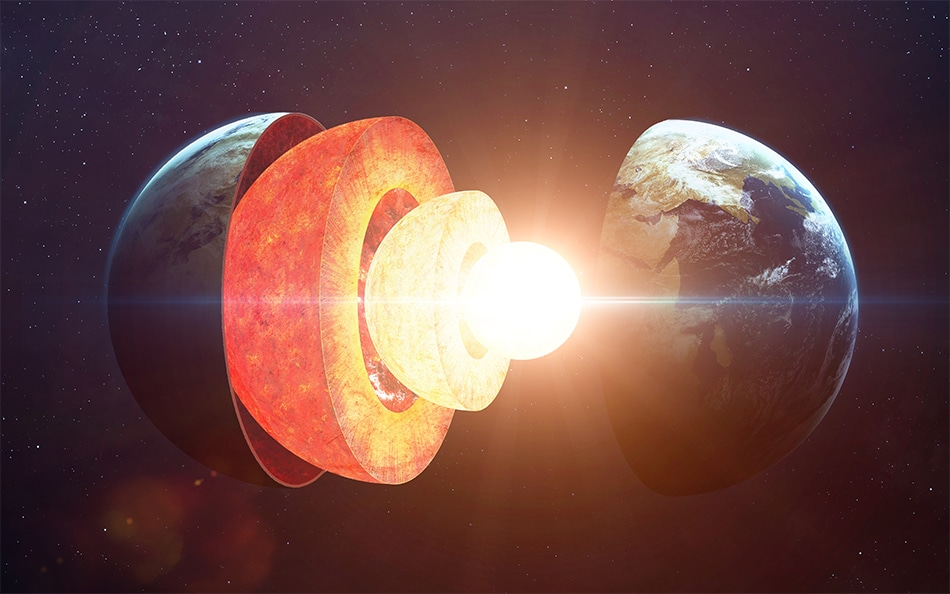# Inner structure of the Earth can be revealed by mathematicsResearchers claim to have found a solution to an open problem in differential geometry: the boundary-rigidity conjecture (BRC).

If verified, it will be a breakthrough in the field and for applications such as reconstruction of the internal structure of objects, such as seismology and medical imaging.

## The boundary-rigidity conjecture and the reconstruction of an inner structure

The boundary-rigidity conjecture is a mathematical problem in the branch of Riemannian geometry. Crudely speaking, this modern geometry describes curved spaces and its generalization was the basis of Einstein's general theory of relativity.

Researchers already know how to determine the shortest path between two points in a Riemannian manifold (curved space) by the way that the curvature varies. However, the boundary-rigidity conjecture is the verification of whether the opposite is also valid: can the curvature be determined when the length of the shortest path between two points on a boundary is known?

Therefore the boundary-rigidity conjecture can be understood as the problem of how to reconstruct the inner structure of an object, from the distances between each pair of boundary points contained in a Riemannian (curved space) structure. By measuring the time travelled and velocity of waves inside an object, the shortest path could be found and then the overall structure could be resolved.

Stefanov, Uhlmann, and Vasy are the three mathematicians who's research is now being checked by other researchers, have been working on this problem since the 1990s, and although they have solved the two-dimensional problem (curved surfaces), the conjecture still doesn’t have the solutions to spatial and other dimensions.

One issue that must be worked is that the conjecture assumes a continuous space, that is, every point in the space of study should be collected – but the experimental data are sets of discrete points, obtained at dispersed locations. However, this can be overcome by numerical methods and computer simulations.

According to an article published in the Nature News, Maarten de Hoop - a computational seismologist at Rice University in Houston (Texas) – stated that the result probably won’t change much of the overall picture that we already have of Earth’s structure. However, it would give us additional information on known mechanisms, “such as the mantle plumes underneath Iceland or Hawaii - and perhaps, the discovery of new ones’’.

## How BRC would reveal Earth's inner structure

The imaging of the internal structure of the Earth is performed using signals that can get from the inner shells to the surface: these signals are the seismic waves. Seismic waves travel through the Earth's inner shells with different speeds accordingly to the properties of the geophysical medium. They can be caused by earthquakes, volcanic eruptions, powerful explosions due to human activity, large landslides, etc. Due to the Earth’s geometric characteristics, wave paths are not straight, but indeed they are curved lines.

Seismic stations around the world record the seismic waves that arrive in their locations and also the time it takes for the waves to reach them. As the velocity of those waves depends on the inner geometric structure of the Earth,  BRC solution would make use of this information to recover the latter.

1. Stefanov, P., Uhlmann, G. & Vasy, A. Preprint at https://arxiv.org/abs/1702.03638 (2017).
2. Castelvecchi, D. Nature 542, 281–282 (February 2017) doi:10.1038/nature.2017.21439
3. Geometric Inverse problems and Applications. Available at: https://www.helsinki.fi/en/researchgroups/inverse-problems/research/geometric-inverse-problems-and-applications
4. How does a seismic station work? Available at: http://www.usarray.org/public/about/how